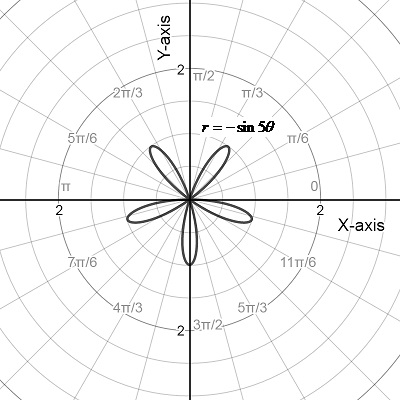Chapter 10.4, Problem 74E

Chapter
Section
Textbook Problem

# Tangent Lines at the Pole In Exercises 73-80, sketch a graph of the polar equation and find the tangent line(s) at the pole (if any). r = − sin 5 θ

To determine

To graph:

Sketch a graph of the polar equation and then find the tangent line at the poleExplanation

Given: The polar equation is r=sin5θ

Graph is given below:

The given polar equation is r=sin5θ

The curve generates when θ changes from [0,π]

Now consider

r=f(θ)=sin5θ

Differentiate with respect to

θ,

f'(θ)=5cos5θ

Now f(θ)=0

sin5θ=0

θ=0,π5,2π5

Thus, <

### Still sussing out bartleby?

Check out a sample textbook solution.

See a sample solution

#### The Solution to Your Study Problems

Bartleby provides explanations to thousands of textbook problems written by our experts, many with advanced degrees!

Get Started

#### Expand each expression in Exercises 122. (2x3)2

Finite Mathematics and Applied Calculus (MindTap Course List)

#### Find the median for the following set of scores: 1, 9, 3, 6, 4, 3, 11, 10

Essentials of Statistics for The Behavioral Sciences (MindTap Course List)

#### In Problems 35-42, simplify each complex fraction. 41.

Mathematical Applications for the Management, Life, and Social Sciences

#### Proof Prove that u(vw)=(uw)v(uv)w

Calculus: Early Transcendental Functions (MindTap Course List)

#### The range of is: (−∞,∞) [0, ∞) (0, ∞) [1, ∞]

Study Guide for Stewart's Multivariable Calculus, 8th

#### True or False: converges absolutely.

Study Guide for Stewart's Single Variable Calculus: Early Transcendentals, 8th